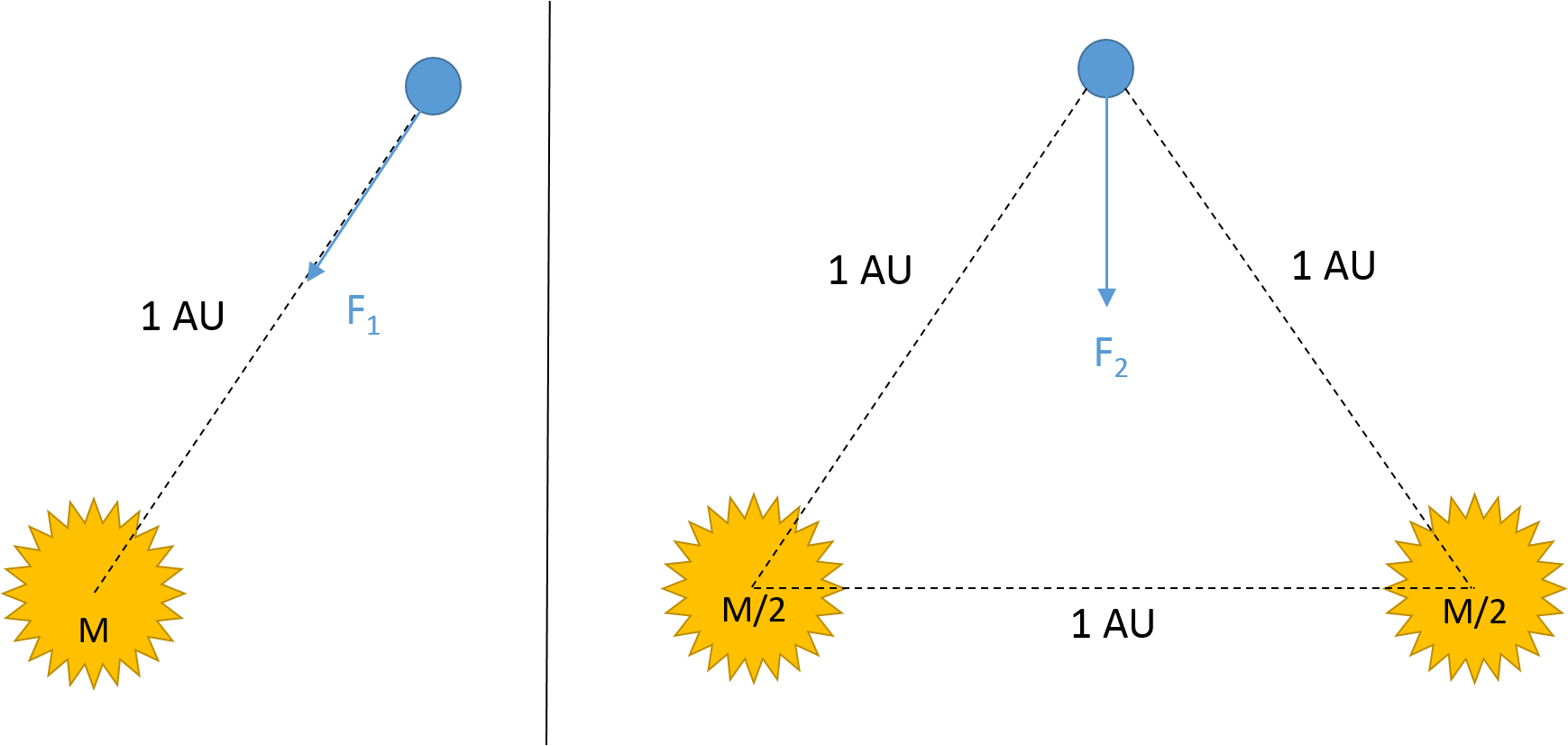# Splitting The Sun In TwoLet's imagine the Earth orbiting two stars that are separated by a distance of $1 \text{ AU}$, each with half the mass of the Sun. When the distance between the Earth and both stars is $1 \text{ AU}$, the net gravitational force acting on Earth is $F_2$.

If the gravitational force applied by the Sun on the Earth at an orbital distance of $1 \text{ AU}$ is $F_1$. What is $\dfrac{F_2}{F_1}$?

Note: $1 \text{ AU}$, which stands for "Astronomical Unit," is the actual average orbital distance between Sun and Earth.

×